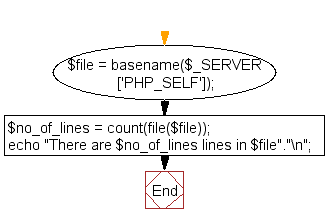﻿ PHP Exercise: Count number of lines in a file - w3resource

# PHP Exercises : Count number of lines in a file

## PHP : Exercise-16 with Solution

Write a PHP script to count number of lines in a file.

Note : Store a text file name into a variable and count the number of lines of text it has.

Sample Solution: -

PHP Code:

``````<?php
\$file = basename(\$_SERVER['PHP_SELF']);
\$no_of_lines = count(file(\$file));
echo "There are \$no_of_lines lines in \$file"."\n";
?>
``````

Sample Output:

```There are 5 lines in a6924e70-5a4c-11e7-b47b-99347412a245.php
```

Flowchart:basename() function: The basename(path,suffix) function is used to get the filename from a path.

count() function: The count() function is used to count the elements of an array or the properties of an object.

Note: For objects, if you have SPL installed, you can hook into count() by implementing interface Countable. The interface has exactly one method, Countable::count(), which returns the return value for the count() function.

PHP Code Editor:

Have another way to solve this solution? Contribute your code (and comments) through Disqus.

What is the difficulty level of this exercise?

Test your Programming skills with w3resource's quiz.

﻿

## PHP: Tips of the Day

Mutates the original array to filter out the values specified

Example:

```<?php
function tips_pull(&\$items, ...\$params)
{
\$items = array_values(array_diff(\$items, \$params));
return \$items;
}

\$items = ['x', 'y', 'z', 'x', 'y', 'z'];
print_r(tips_pull(\$items, 'y', 'z'));
?>
```

Output:

```Array
(
 => x
 => x
)
```